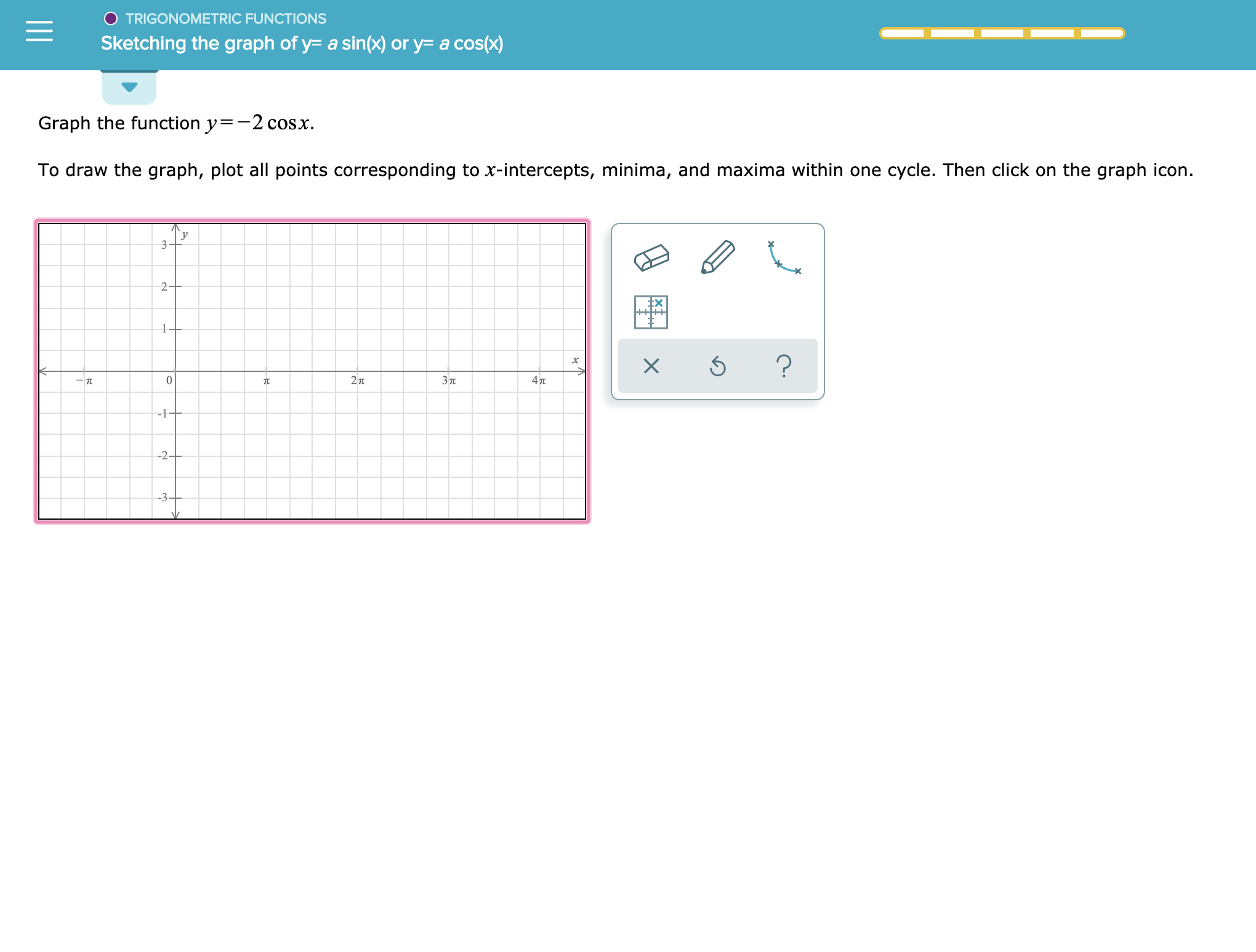TRIGONOMETRIC FUNCTIONSSketching the graph of y- a sin(x) or y= a cos(x)Graph the function y-2 cosxTo draw the graph, plot all points corresponding to x-intercepts, minima, and maxima within one cycle. Then click on the graph icon.32F1?27TЗп4Tt-1+-2+-3+

Question

See attachmenthelp_outlineImage TranscriptioncloseTRIGONOMETRIC FUNCTIONS Sketching the graph of y- a sin(x) or y= a cos(x) Graph the function y-2 cosx To draw the graph, plot all points corresponding to x-intercepts, minima, and maxima within one cycle. Then click on the graph icon. 3 2F 1 ? 27T Зп 4Tt -1+ -2+ -3+ fullscreen
Step 1

Calculation:

The x intercepts of y = −2cos(x) is [(π/2) + 2πn,0] and [(3π/2) + 2πn,0], n =0,1,2,3…

The y-intercept is (0, −2).

The maximum point is...

Want to see the full answer?

See Solution

Want to see this answer and more?

Our solutions are written by experts, many with advanced degrees, and available 24/7

See Solution
Tagged in

Other